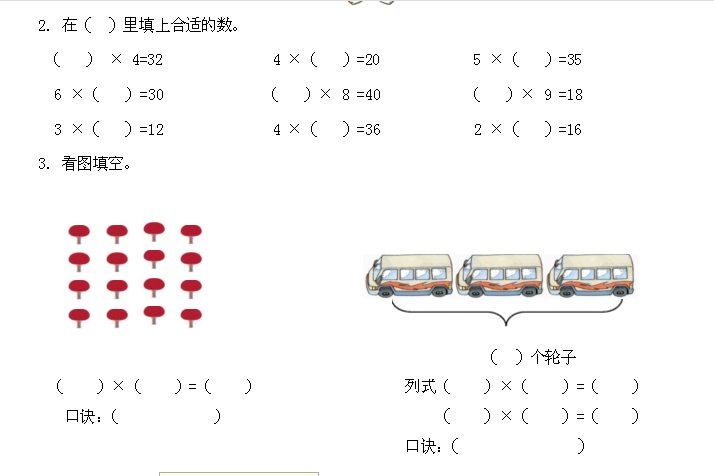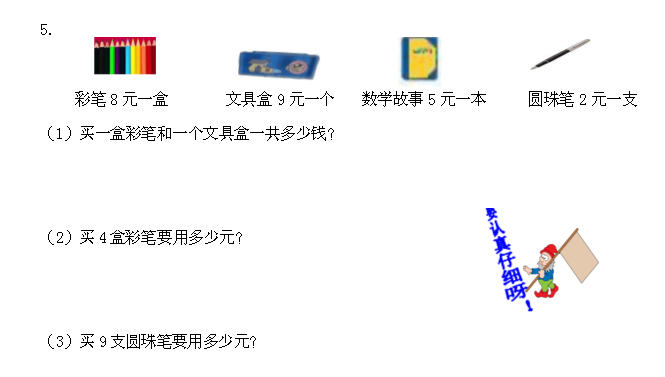## 5.6回家路上练习题及答案【北师大版】

《回家路上》课时练

1. 计算

3×4= 5×4= 5×9= 2×9= 2×8= 9×4=

4×7= 8×4= 2×5= 5×5= 5×6= 4×4=

1. 在（）里填上合适的数。

（）×4=32 4×（）=20 5×（）=35

6×（）=30 （）×8=40 （）×9=18

1. 看图列式计算

（1）（）×（）=（）

（2）（ ）个轮子

（）×（）=（）

4.1. 买一盒彩笔和一个文具盒一共多少钱？
2. 买4盒彩笔要用多少元？
3. 买9支圆珠笔要用多少元？

1. 12 20 45 18 16 36；28 32 10 25 30 16
2. 8,5,7；5,5,2
3. 4×4=16 四四十六 12 4×3=12 三四十二
4. （1） 8+9=17（元） （2）4×8=32（元） （3）2×9=18（元）